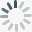×
BigML is working hard to support a wide range of browsers. Your experience will be better with:
Prediction Explanation
Apr2018For any classification or regression model, you can now request an explanation for your predictions. The explanation represents the most important factors considered by the model in the prediction given the input values. Therefore, each input value will have an associated importance where the importances across all input values sum up to 100%.

While the prediction explanation for decision tree models is calculated using the prediction path, for other models (ensembles, logistic regression, and deepnets) the prediction explanation is calculated by aggregating the results of over a thousand distinct predictions that use random perturbations of the input data.

To read more about the prediction explanation with the BigML Dashboard please read the sections "Prediction Explanation" of the Classification and Regression document. To get the prediction explanation with the BigML API you need to use the "explain":true argument as detailed in API documentation.

supervised classification regression single predictions field importances prediction
New voting strategies for ensembles
Jan2018As you may know, ensembles are composed of several decision trees. For non-boosted ensembles, each single tree returns a prediction given the input data. These predictions need to be combined so the ensemble returns a single prediction for each new instance.

For the previous years, BigML has been offering what we called "combiners": plurality, confidence weighted and probability weighted. Each of them provided a different strategy to combine single tree predictions. Now BigML is improving these strategies for ensembles and homogenizing the predictions across all resources, offering three new options that will replace our old combiners forever:

• Probabilities: Averages the per-class probability distributions for all trees in the ensemble and predicts the class with the highest probability. For regression ensembles, the global prediction is the mean of the individual predictions.
• Confidences: Averages the per-class confidence distributions for all trees in the ensemble and predicts the class with the highest confidence. For regression ensembles, the global prediction is the mean of the individual predictions weighted by the expected error.
• Votes: Gives one vote to each model in the ensemble. For classification models, the category with the majority of votes wins. For regression models, the global prediction is the mean of the individual predictions (it gives the same results as the probability strategy).

You can find these new options among the prediction arguments in the BigML API under the name of operating_kinds. The old combiners will also still be available from the API for a while.

probabilities confidences votes evaluations batch predictions ensembles decision forests combiners prediction
Operating thresholds for Single Predictions
Jan2018Set a threshold for the positive class when creating single predictions for any classification model. This technique is very useful for imbalanced datasets where one or a few classes are the majority classes. In these cases, models tend to predict the majority classes at the expense of the minority class that is usually the class of interest (called the positive class).

By setting a threshold for the positive class, this class will be predicted if its probability (confidence or votes depending on the type of measure you select) is greater than the established threshold, otherwise the following class with the highest probability (confidence or votes) will be predicted instead.

Whether you build a single decision tree, an ensemble, a logistic regression or a deepnet, you can select a positive class and set a threshold for a given prediction.

predictions probabilities confidences thresholds positive class imbalanced prediction miscellaneous
Deepnet Predictions
Oct2017One of the main goals of any BigML resource is making predictions, and Deepnets are no exception. Deepnets can be used to predict categorical or numeric values. As Deepnets have more than one layer of nodes between the input and the output layers, the output will be the network’s prediction. In the case of categorical objective fields, an array of per-class probabilities will be returned, while a single, real value will be predicted for regression problems. You can perform single predictions, if you want to predict just one instance; or batch predictions if you want to predict multiple instances at the same time.

deepnets classification regression probabilities prediction
Batch Field Importances
Dec2016This feature enables you to include the field importances in your batch predictions, i.e., a set of percentages indicating how much each field in your dataset contributed to the prediction of a given instance. You can include those values in your output file and dataset either with BigML Dashboard or the API. This will give you a better understanding of your predictions as it will reveal which are the most relevant fields factoring in a given prediction.

supervised predictions batch predictions regression classification models ensembles api dashboard prediction
Online predictions
Jul2014New client-side predictions make it easier than ever to explore the influence of each field in your models, ensembles or clusters. In addition, we are open sourcing the related Javascript libraries so you can leverage this functionality to build very powerful and dynamic apps and web services.

free client-side javascript summer2014 prediction
Sending Request...
Sending Request...